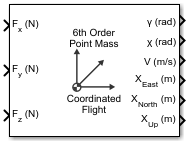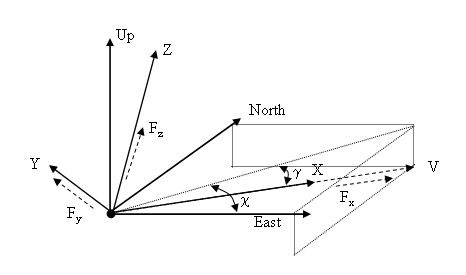Documentation

# 6th Order Point Mass (Coordinated Flight)

Calculate sixth-order point mass in coordinated flight

## Library

Equations of Motion/Point Mass## Description

The 6th Order Point Mass (Coordinated Flight) block performs the calculations for the translational motion of a single point mass or multiple point masses.The translational motion of the point mass [XEast XNorth XUp]T are functions of airspeed (V), flight path angle (γ), and heading angle (χ),

`$\begin{array}{l}{F}_{x}=mV\\ {F}_{y}=\left(mV\mathrm{cos}\gamma \right)\stackrel{˙}{\chi }\\ {F}_{z}=mV\stackrel{˙}{\gamma }\\ {\stackrel{˙}{X}}_{East}=V\mathrm{cos}\chi \mathrm{cos}\gamma \\ {\stackrel{˙}{X}}_{North}=V\mathrm{sin}\chi \mathrm{cos}\gamma \\ {\stackrel{˙}{X}}_{Up}=V\mathrm{sin}\gamma \end{array}$`

where the applied forces [Fx Fy Fh]T are in a system is defined by x-axis in the direction of vehicle velocity relative to air, z-axis is upward, and y-axis completes the right-handed frame, and the mass of the body m is assumed constant.

## Parameters

Units

Specifies the input and output units:

Units

Forces

Velocity

Position

`Metric (MKS)`

Newton

Meters per second

Meters

`English (Velocity in ft/s)`

Pound

Feet per second

Feet

`English (Velocity in kts)`

Pound

Knots

Feet

Initial flight path angle

The scalar or vector containing initial flight path angle of the point mass(es).

Initial heading angle

The scalar or vector containing initial heading angle of the point mass(es).

Initial airspeed

The scalar or vector containing initial airspeed of the point mass(es).

Initial downrange [East]

The scalar or vector containing initial downrange of the point mass(es).

Initial crossrange [North]

The scalar or vector containing initial crossrange of the point mass(es).

Initial altitude [Up]

The scalar or vector containing initial altitude of the point mass(es).

Initial mass

The scalar or vector containing mass of the point mass(es).

## Inputs and Outputs

InputDimension TypeDescription

First

Contains the force in x-axis in selected units.

Second

Contains the force in y-axis in selected units.

Third

Contains the force in z-axis in selected units.
OutputDimension TypeDescription

First

Contains the flight path angle in radians.

Second

Contains the heading angle in radians.

Third

Contains the airspeed in selected units.

Fourth

Contains the downrange or amount traveled East in selected units.

Fifth

Contains the crossrange or amount traveled North in selected units.

Sixth

Contains the altitude or amount traveled Up in selected units.

## Assumptions and Limitations

The block assumes that there is fully coordinated flight, i.e., there is no side force (wind axes) and sideslip is always zero.

The flat flat Earth reference frame is considered inertial, an excellent approximation that allows the forces due to the Earth's motion relative to the “fixed stars” to be neglected.

#### Introduced before R2006a

##### Support평가판 신청